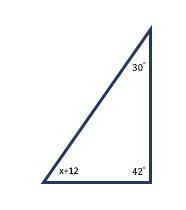# Triangle Sum Theories

Now, using this information, can you determine if these angle measures will create one unique triangle or many triangles?

If you use a protractor to draw triangles with these measures, you would see that it is possible to draw many different triangles and they would all be similar. The side lengths would be proportional and the angles would be congruent.

There are other theorems used to construct unique triangles.

• SAS – Side, Angle, Side (This theorem involves two sides and the included angle.)
• ASA- Angle, Side, Angle (This theorem has 2 angles and the side between them)
• SSS – Side, Side, Side (This theorem has 3 sides with specified lengths that meet the triangle inequality theorem.)

Time for a short video demonstrating the Angle Sum Theorem.It is time to use the Angle Sum Theorem to solve for the missing angle in a triangle.

Look at the triangle below. We know the measurements of two of the angles, but the third one is a mystery. How can we use the Triangle Angle Sum Theorem to find the measurement of that third angle?The Triangle Angle Sum Theorem states that the sum of all angles in a triangle equal 180°

You can write an equation to solve for the missing side:

86 + 53 + x = 180

139 + x= 180
-139        -139

x = 41

41 + 86 + 53 = 180

The missing angle = 41°

Here is another type of problem you can solve using this theorem. Instead of solving for the missing angle, solve for x.Write an equation using the triangle angle sum theorem.

X + 12 + 32 + 40= 180 (Combine terms)

X + 84 = 180
-84   – 84

x = 96 If you plug this into the angle, you can also use x to identify the measure of the angle!

(source)